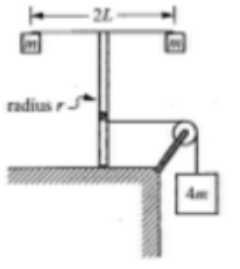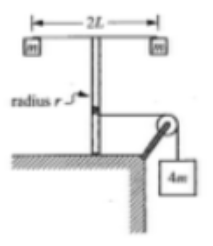# Problem: A light string that is attached to a large block of mass 4m passes over a pulley with negligible rotational inertia and is wrapped around a vertical pole of radius r, as shown above. The system is released from rest, and as the block descends the string unwinds and the vertical pole with its attached apparatus rotates. The apparatus consists of a horizontal rod of length of length 2L, with a small block of mass m attached at each end. The rotational inertia of the apparatus is assumed to be 2ml2. (a) If the downward acceleration of the large block is measured to be a, determine the tension in the string, in terms of the acceleration of the falling block, (b) Dtermine the torque exerted on the rotating pole by the string, in terms of the mass of the block and the acceleration. (c) When the large block has descended a distance D, how does the instantaneous rotational kinetic energy of the apparatus compare with the value 4mgD? Check the appropriate space below and justify your answer. ________ Greater than 4mgD                 ____________ Equal to 4mgD                 _______________ Less than 4mgDNow consider the experiment again, this time including the rotational inertia of the small pulley.(d) If the rotational inertia of the pulley were large enough to have an effect on the experiment, would you predict your answers to be different? Explain your response.

###### FREE Expert Solution

(a)

Newton's second law:

$\overline{){\mathbf{\Sigma }}{\mathbf{F}}{\mathbf{=}}{\mathbf{m}}{\mathbf{a}}}$, where ΣF is the sum of forces, m is mass, and a is acceleration.

Now we have:

4mg - T = 4ma

88% (46 ratings)###### Problem DetailsA light string that is attached to a large block of mass 4m passes over a pulley with negligible rotational inertia and is wrapped around a vertical pole of radius r, as shown above. The system is released from rest, and as the block descends the string unwinds and the vertical pole with its attached apparatus rotates. The apparatus consists of a horizontal rod of length of length 2L, with a small block of mass m attached at each end. The rotational inertia of the apparatus is assumed to be 2ml2

(a) If the downward acceleration of the large block is measured to be a, determine the tension in the string, in terms of the acceleration of the falling block,

(b) Dtermine the torque exerted on the rotating pole by the string, in terms of the mass of the block and the acceleration.

(c) When the large block has descended a distance D, how does the instantaneous rotational kinetic energy of the apparatus compare with the value 4mgD? Check the appropriate space below and justify your answer.

________ Greater than 4mgD                 ____________ Equal to 4mgD                 _______________ Less than 4mgD

Now consider the experiment again, this time including the rotational inertia of the small pulley.(d) If the rotational inertia of the pulley were large enough to have an effect on the experiment, would you predict your answers to be different? Explain your response.